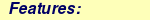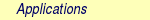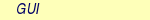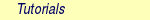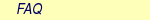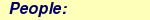MOLCAS manual:Next: 8.3 caspt2 Up: 8. Programs Previous: 8.1 alaska

Subsections

# 8.2 averd

## 8.2.1 Description

Averd computes average densities and corresponding natural molecular orbitals. Given a set of density matrices in the same basis set, an average density matrix is constructed and diagonalized to give average natural orbitals. These orbitals have non-integer occupation numbers, although they usually are fairly well clustered in one strongly occupied part and one weakly occupied part. From basic mathematical properties of natural orbitals, a truncated set of the orbitals constructed this way constitutes the most compact basis of one-electron functions of that given size. In other words, the smallest set of functions to span the average space within a certain accuracy has been obtained. Averd is in essence very similar to Genano.

## 8.2.2 Dependencies

Averd needs a set of input densities. Any of the programs, which generate a density must preceed.

## 8.2.3 Files

Below is a list of the files that are used/created by Averd.

### 8.2.3.1 Input files

 File Contents RUNFILE File for communication of auxiliary information generated by the program SEWARD. ONEINT File with one-electron integrals generated by SEWARD. RUN*** A set of RunFiles on which a density matrix is stored. This density matrix is read and added to the average. If ORBItals is given, these files are not needed. NAT*** A set of orbitals in the format generated by SCF or RASSCF. The orbitals are used to generate a density matrix, which is added to the average. Only required if ORBItals is given.

### 8.2.3.2 Output files

 File Contents AVEORB The average orbitals generated by Averd. They are stored in the same way as SCF-orbitals and can be used as INPORB.

## 8.2.4 Input

 Keyword Meaning TITLe Title of the calculation. WSET Followed by two rows. On the first the number of input orbitals are given, N. Second row contains N numbers each giving a weight for the kth input density matrix to the average density. The weights are normalized by Averd, hence only the ratio of the numbers have any significance. This keyword is mandatory. PRINt Print level. 1 is default. Higher than 3 is not recommended for the average user. ORBItals This keyword signifies that the densities should be created from average orbitals in the files NAT***, not directly from the density matrices on the files RUN***. The default is to use the density matrices on RUN***. OCCUpation Followed by one number. The number of average orbitals with an occupation higher than this number is reported for each symmetry. Since the occupation is the guide for how to truncate the orbitals in subsequent applications, this is an easy way to get hold of that number. The default is 10-5.

### 8.2.4.1 Input example

In this example, two density matrices are averaged and their average orbitals are computed.

&Seward
Basis  set
O.ano-s.Pierloot.10s6p3d.7s4p2d.
O  0.0000  0.0000  0.3000
End  of  Basis  set
Basis  set
H.ano-s.Pierloot.7s3p.4s1p.
H1  0.0000  -1.4300  -0.8070
H2  0.0000  1.4300  -0.8070
End  of  Basis  Set

&FfPt
Dipo
z  0.005
End  of  Input

&Scf
Occupation
5
>>COPY  \$Project.RunFile  RUN001

&FfPt
Dipo
z  -0.005

&Scf
Occupation
5
End  of  Input
>>COPY  \$Project.RunFile  RUN002

&Averd  &End
Wset
2
1.0  1.0
Occupation
1d-6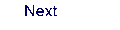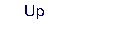Next: 8.3 caspt2 Up: 8. Programs Previous: 8.1 alaska